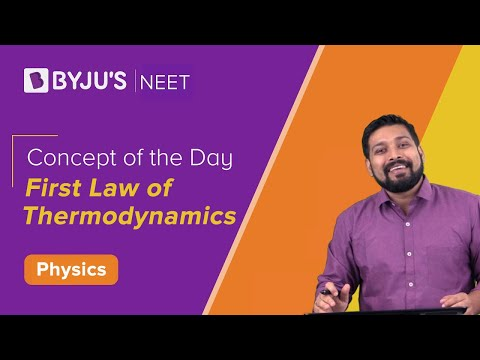# Boltzmann Equation

## What is Boltzmann Equation?

The Boltzmann equation or Boltzmann transport equation (BTE) explains the behaviour of a fluid with temperature. It also explains the change of a macroscopic quantity in a thermodynamic system, such as energy, charge or particle number.

The Boltzmann equation is given as:

 $$\begin{array}{l}\frac{P_{Sb}}{P_{Sa}}=\frac{N_{b}}{N_{a}}=\frac{gbe^-{\frac{Eb}{kT}}}{ga^-\frac{Ea}{kT}}=\frac{gb}{ga}e^-\frac{(Eb-Ea)}{kT}\end{array}$$

## Ludwig Boltzmann

Ludwig Boltzmann was a great Physicist and a Philosopher of the 19th century. He has developed the statistical explanation of the second law of thermodynamics and has contributed to the development of statistical mechanics. Boltzmann’s kinetic theory of gas was also one of the significant contributions.

The Boltzmann equation, also known as the Boltzmann transport equation (BTE), is used to study the statistical behaviour of the thermodynamic system which is not in a state of equilibrium. It was devised in the year 1872 by Ludwig Boltzmann.

## Applications of Boltzmann equation

• Conservation equations: Boltzmann equation is used in the derivation of conservation laws of mass, momentum, charge, and energy which are part of fluid dynamics.
• Hamiltonian mechanics: Classical mechanics was reformulated as Hamiltonian mechanics with the help of different mathematical formulations.
• Quantum theory and violation of particle number: Quantum Boltzmann equation is used to find out the number of particles that are not conserved in the collisions which are widely used in physical cosmology.
• General relativity and astronomy: Boltzmann equation finds application in galactic dynamics.

### Boltzmann Equation Examples

Example 1:

Determine the temperature at which the number of hydrogen atoms is equal in the ground state when n = 1 and the second excited state is n = 3. Also, the required energy is E3 = -1.5 eV.

Solution:

Given:

Ground state, n = 1

Excited-state, n = 3

The required energy, E3 = -1.5 eV

Using the Boltzmann equation,

N3/N1 = 1 = 18/2 e-12.1/kT

kT = -(12.1/ln 0.111) = 5.51 eV

T = 5.51 x 40 x 300 = 66,000 K

However, we know that for N1 = N2, the required temperature is 85,400 K

For this temperature, kT = 7.12 eV

Therefore,

N3 = N1 18/2 e-12.1/7.12

N3 = 1.64 N1

Related Physics articles

Visit BYJU’S and learn more about Stefan Boltzmann law through engaging videos. Also, register to “BYJU’S – The Learning App” for loads of interactive, engaging Physics-related videos and an unlimited academic assist.

## Watch the video and learn how the system of particles behaves when work, heat, temperature, and pressure are involved.## Frequently Asked Questions – FAQs

Q1

### What is the Boltzmann equation also known as?

The Boltzmann equation is also known as the Boltzmann transport equation.
Q2

### Who put forth the Boltzmann equation?

In 1872, the great physicist and philosopher Ludwig Boltzmann put forth the Boltzmann equation.

Q3

### Give the Boltzmann equation.

The Boltzmann equation is given by:
$$\begin{array}{l}\frac{P_{Sb}}{P_{Sa}}=\frac{N_{b}}{N_{a}}=\frac{gbe^-{\frac{Eb}{kT}}}{ga^-\frac{Ea}{kT}}=\frac{gb}{ga}e^-\frac{(Eb-Ea)}{kT}\end{array}$$
Q4

True.
Q5

### What are the applications of the Boltzmann equation?

Boltzmann equation is used in:
• Derivation of conservation equations
• Hamiltonian mechanics
• Quantum theory and violation of particle number
• General relativity and astronomy
• Test your knowledge on Boltzmann Equation